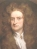Physics!What is physics discussion doing on a website about skiing? Skiing and physics have a lot to do with each other. Of course when skiing, no one thinks about force and velocity vectors but since we are trying to control physical forces it can only be helpful to understand those forces and how they all come into play while skiing.

I know, the guy pictured up above doesn’t look like Einstein. You are right, he isn’t Albert Einstein he is Sir Isaac Newton. The physics and math developed by Sir Isaac Newton are the physics and math required to understand the interplay of velocity, gravity, and forces at work while skiing.

If you ski near the speed of light (3×108 meters/second or 675,000,000 miles/hour) then you need Einstein’s physics, but I suspect you ski slow enough relativity doesn’t come into play with your skiing. So we honor Sir Isaac Newton instead of Einstein.

Sir Isaac Newton established the fundamentals of understanding the forces that come into play while skiing. He established the formula that tells how strong the force of gravity pulls on an object and three laws of motion.

Newton’s Three Laws of Motion.

1. Every body persists in its state of rest or of uniform motion in a straight line unless it is compelled to change that state by forces impressed on it. (page 59 Fundamentals of Physics Second Edition by Halliday and Resnick).
2. This means a body in motion keeps going straight until some other force causes that body to slow down, speed up, [ed. note – I changed the language here] or to change the direction of its motion. Similarly, a body not moving will not move unless some force causes it to.

3. F=ma. Force is equivalent to the mass times the acceleration, (force and acceleration are vector quantities, that is they have a direction as well as a size).
4. To every action there is always opposed an equal reaction; or, the mutual actions of two bodies upon each other are always equal, and directed to contrary parts. (page 65 Fundamentals of Physics Second Edition by Halliday and Resnick).
5. That is to say, if you push on something it pushes back equally hard.

Those three laws along are the fundamental laws you need to understand the forces at play while skiing. Actually, I fib a bit as we also have to deal with friction and wind resistance, but once we come up with a mathematical way to describe those forces, we can plug them right into Newton’s laws.

Sources:

Good Stuff!

This site uses Akismet to reduce spam. Learn how your comment data is processed.